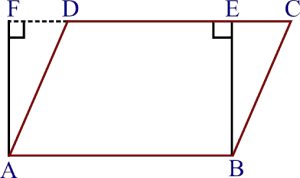Guru

# Parallelogram ABCD and rectangle ABEF are on the same base AB and have equal areas. Show that the perimeter of the parallelogram is greater than that of the rectangle. Q.1

• 0

Give me the best and simple way for solving the question of class 9th of Areas of parallelograms and triangles chapter of math of question no.1 how i solve this question in easy and simple way because it is very important type of question Parallelogram ABCD and rectangle ABEF are on the same base AB and have equal areas. Show that the perimeter of the parallelogram is greater than that of the rectangle.

Share

1.Given,

|| gm ABCD and a rectangle ABEF have the same base AB and equal areas.

To prove,

Perimeter of || gm ABCD is greater than the perimeter of rectangle ABEF.

Proof,

We know that, the opposite sides of a|| gm and rectangle are equal.

, AB = DC [As ABCD is a || gm]

and, AB = EF [As ABEF is a rectangle]

, DC = EF … (i)

Adding AB on both sides, we get,

⇒AB + DC = AB + EF … (ii)

We know that, the perpendicular segment is the shortest of all the segments that can be drawn to a given line from a point not lying on it.

, BE < BC and AF < AD

⇒ BC > BE and AD > AF

⇒ BC+AD > BE+AF … (iii)

Adding (ii) and (iii), we get